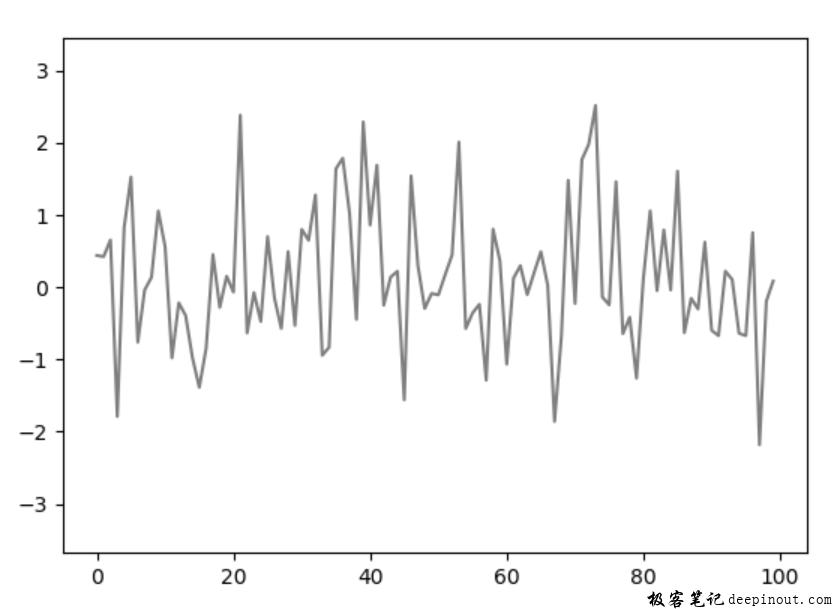# Matplotlib ArtistAnimation方式创建动画

`matplotlib.animation.ArtistAnimation(fig, artists, *args, **kwargs)`

• 第一个参数fig是绘图用的画布，主要用来显示图像和缩放大小。

• 第二个参数artists是表示每个帧绘图数据显示的对象列表，程序里会遍历列表，每一项生成一帧动画帧。

• 第三个参数interval是每帧延时时间，与前面的回调函数一样的。

• 第四个参数repeat_delay是表示重复播放动画之间中的延时，默认是0毫秒。

• 第五个参数repeat是表示是否播放动画完之后重复播放，当设置True时表示重复播放，默认为重复播放。

• 第六个参数blit是表示是否使用优化机制，与前面回调函数的意义一样。

``````ims = []
for i in range(20):
rand = np.random.randn(100) # 100个随机数生成
im = ax.plot(rand) # 显示100数据
ims.append(im) # 保存到列表
``````

`anim = animation.ArtistAnimation(fig, ims, interval=100)`

``````FFwriter = animation.FFMpegWriter(fps=20, extra_args=['-vcodec', 'libx264'],
anim.save('ani-054.mp4', writer = FFwriter)
````````````import numpy as np
from matplotlib import pyplot as plt
from matplotlib import animation

plt.rcParams['animation.ffmpeg_path'] = r'D:\ffmpeg-2020-12-20\bin\ffmpeg.exe'

# 创建绘图轴对象
fig, ax = plt.subplots()

# 生成动画绘制所有数据
ims = []
for i in range(20):
rand = np.random.randn(100)     # 100个随机数生成
im = ax.plot(rand)             # 显示100数据
ims.append(im)                  # 保存到列表

anim = animation.ArtistAnimation(fig, ims, interval=100)

#保存的动画视频文件名为当前文件夹下的ani-054.mp4，帧率为20帧每秒，格式为MP4。
FFwriter = animation.FFMpegWriter(fps=20, extra_args=['-vcodec', 'libx264'],